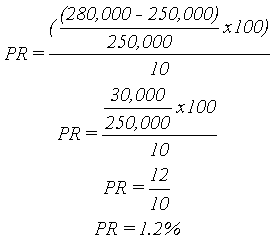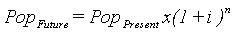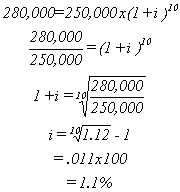Planning Analysis: Calculating Growth Rates

1. Calculating Percent (Straight-Line) Growth Rates

The percent change from one period to another is calculated from the formula:Where:

PR = Percent Rate
VPresent = Present or Future Value
VPast = Past or Present Value

The annual percentage growth rate is simply the percent growth divided by N, the number of years.

Example

In 1980, the population in Lane County was 250,000. This grew to 280,000 in 1990. What is the annual percentage growth rate for Lane County?

VPresent = 280,000
VPast = 250,000
N = 10 YearsThe population of Lane County grew 12 percent between 1980 and 1990 or at an rate of 1.2 percent annually.

2. Calculating Average Annual (Compound) Growth Rates

Another common method of calculating rates of change is the Average Annual or Compound Growth Rate (AAGR). AAGR works the same way that a typical savings account works. Interest is compounded for some period (usually daily or monthly) at a given rate. Here's how it works:

Let's say you had \$100 dollars and invested it in an account that paid 5 percent annually. The table below shows how your interest accumulates.

 Year 0 1 2 3 4 5 Rate \$100 +5% +5% +5% +5% +5% Increase NA \$5 \$5 \$6 \$6 \$6 Amount \$100 \$105 \$110 \$116 \$122 \$128

This can also be written algebraically as:

(((((100 * (1.05) * 1.05) * 1.05) * 1.05) * 1.05)

A. The formula to calculate future population given current population and a growth rate is:Where:

PopPresent = Present Population
i = Growth Rate
n = Number of Periods

To calculate your future balance in the above example the formula would be:

Future Value = \$100 * (1.05)5 = \$128

To perform this on a hand-held calculator take the following steps:

Press 1 + i (growth rate in decimal), the = (equals)

Press yx, then n (the number of periods) <- the compound growth factor

Press * (times) then PopPresent <- the population at the end of n periods or on the calculator:

Press 1 + .05 = 1.05

Press yx, then 5 = 1.28

Press x 100 = \$127.6

B. The formula to calculate a growth rate given a beginning and ending population is:Where:

PopFuture = Future Population
PopPresent = Present Population
i = Growth Rate (unknown)
n = Number of Years

You have to do a little algebraic manipulation to solve for i. Let's use the Lane County example from page 1 to illustrate how this works.

PopFuture = 280,000 (actually 1990)
PopPresent = 250,000 (actually 1980)
i = Growth Rate (????)
n = 10

Therefore:To perform this on a hand-held calculator:

Press N (the number of periods)

Press 1/x

Press X -> M (i.e. store 1/number of periods in memory)

Press PopFuture ¸ PopPresent, the = (equals)

Press yx

Press RM (recall memory), then = (equals)

Press - 1, then = and multiply by 100 to get a percent

Or for our example:

Press 10, then 1/x, then X -> M

Press 280,000 ¸ 250,000, then =

Press yx, then RM, then =

Press - 1, then =, then x 100

You can also use the RATE function in most spreadsheet applications to calculate compound growth rates. In Excel, the basic function is:

=RATE(nper, pmt, pv, fv, type, guess)

Where:

nper - the number of periods (required)

pmt - the payment made each year during the annuity (not required)

pv - present value (required)

fv - future value (required)

type - whether payments occur at the beginning or end of a period (not required)

guess - your best guess of the rate (not required)

Applying this formula to compound growth rates for populations requires some modifications. The required arguments can be thought of as follows:

nper - the number of years during the analysis period

pmt - leave blank

pv - population during earlier period (must be negative)

fv - population during later period

type - leave blank

guess - leave blank

Applying the RATE function to the example on the previous page yields:

=RATE(10,,-250000,280000)